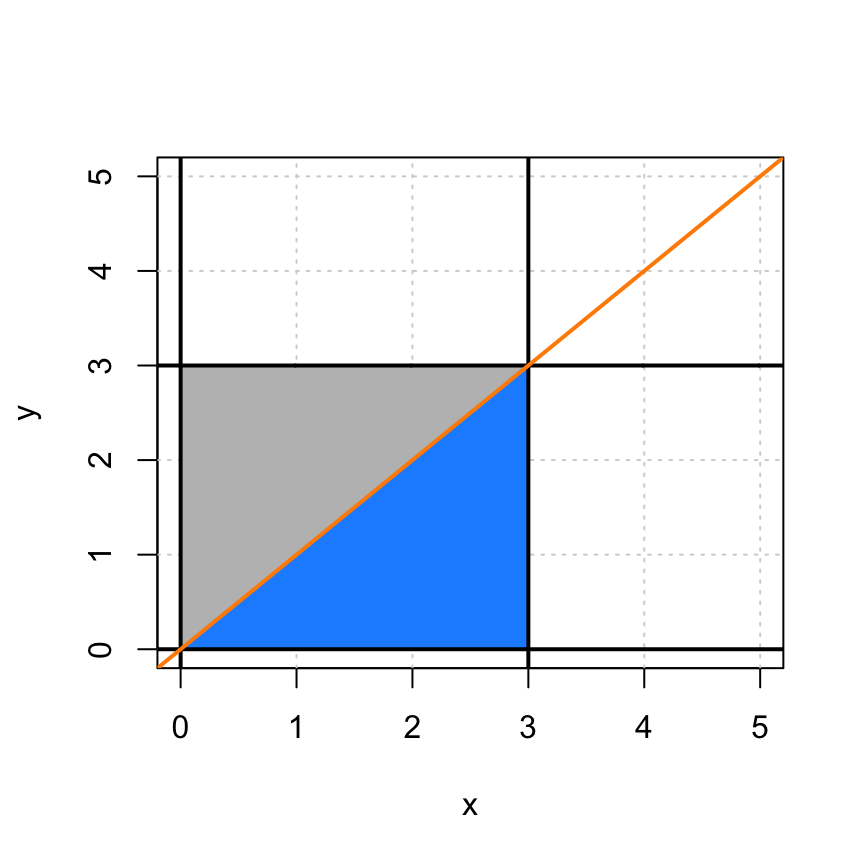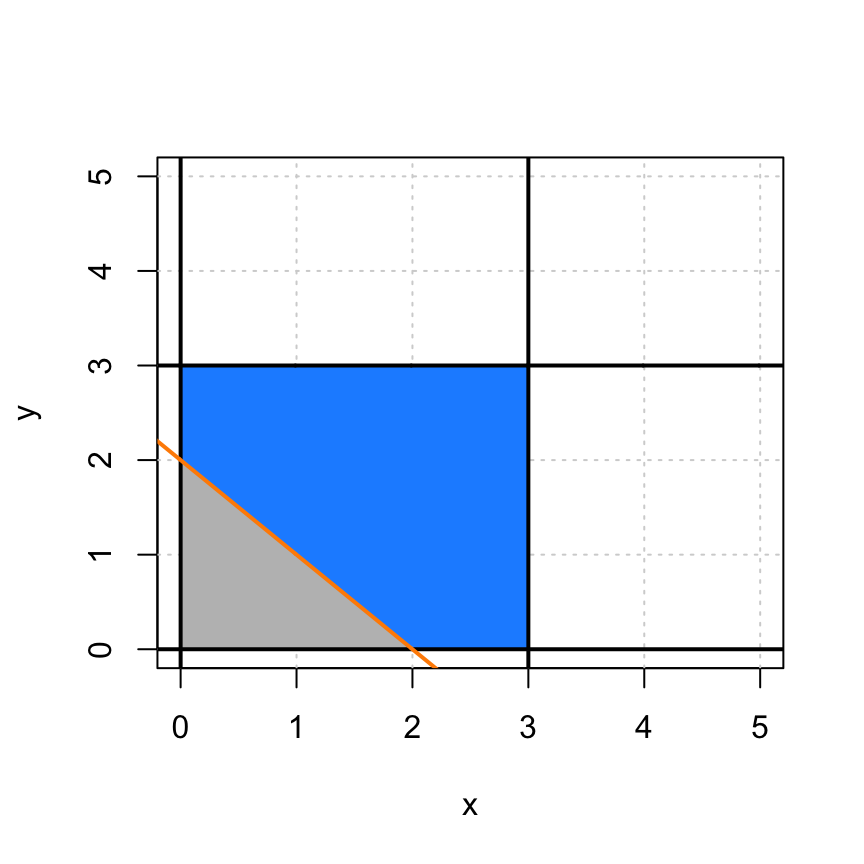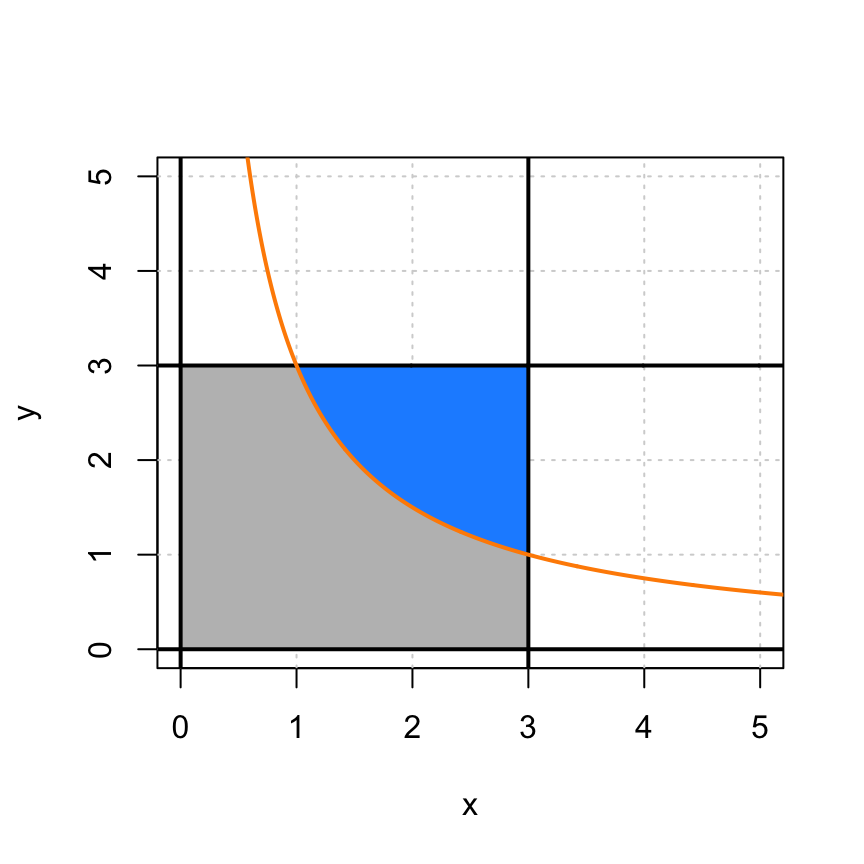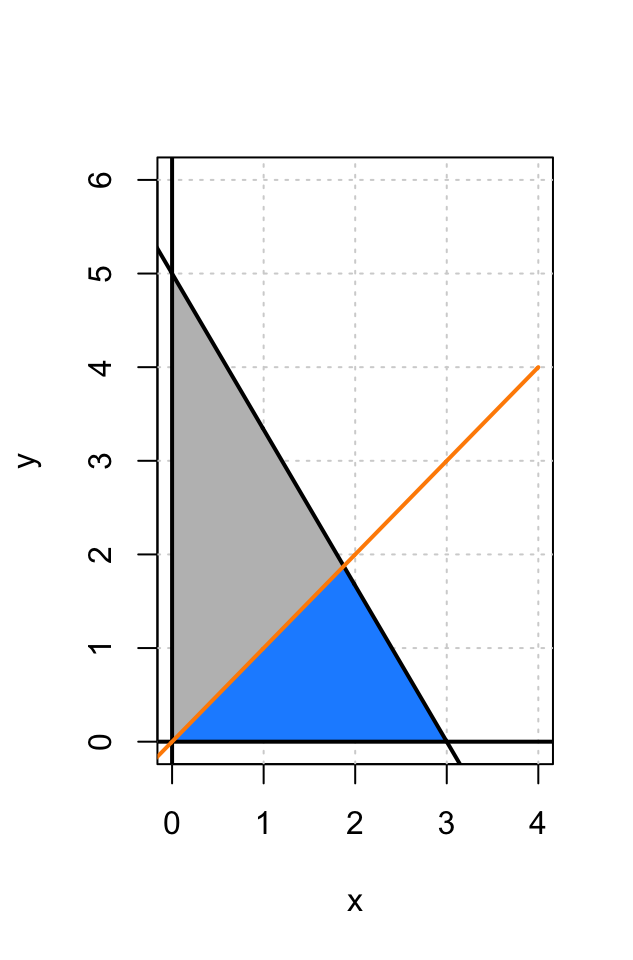## Exercise 1

Let $$H$$ denote the number of times Harry Potter manages to irritate Professor Snape in one day. Let $$R$$ denote the number of times Ron Weasley manages to irritate Professor Snape in one day. Let the joint probability mass function for $$(H, R)$$ be

$f(h, r) = \begin{cases} \displaystyle\frac{h + r}{24} & h \in \{1, 2\}, \ r \in \{0, 1, 2, 3\} \\[1.5ex] \ \ \ 0 &\text{otherwise} \end{cases}$

(a) Calculate $$\text{Cov}[H, R]$$.

Solution:

First, we obtain the marginal distributions of $$H$$ and $$R$$.

$f_H(h) = \sum_{r = 0}^{3} \frac{h + r}{24} = \frac{4h + 6}{24}, \ \ \ h \in \{1, 2\}$

$f_R(r) = \sum_{h = 1}^{2} \frac{h + r}{24} =\frac{2r + 3}{24}, \ \ \ r \in \{0, 1, 2, 3\}$

We can then obtain some intermediate expectations.

$\text{E}[H] = \sum_{h = 1}^{2} h \cdot \frac{4h + 6}{24} = \frac{19}{12}$

$\text{E}[R] = \sum_{r = 0}^{3} r \cdot \frac{2r + 3}{24} = \frac{23}{12}$

$\text{E}[HR] = \sum_{r = 1}^{2} \sum_{h = 0}^{3} hr \cdot \frac{h + r}{24} = 3$

Then finally we have

$\text{Cov}[H, R] = \text{E}[HR] - \text{E}[H] \cdot \text{E}[R] = 3 - \frac{19}{12} \cdot \frac{23}{12} = \boxed{-\frac{5}{144}} \approx \boxed{-0.03472}$

(b) Each day, Professor Snape takes 20 points from Gryffindor, simply because he can. Additionally, Professor Snape takes 10 points from Gryffindor each time Ron Weasley irritates him. The only person that Snape despises more than Ron is Harry, so Professor Snape takes 15 points from Gryffindor each time Harry irritates him. If these are the only three sources of point deductions for Gryffindor, what is the expected point loss for Gryffindor each day?

Solution:

As a result of the linearity of expectation, we have

\begin{aligned} \text{E}[20 + 15H + 10R] &= 20 + 15 \cdot \text{E}[H] + 10 \cdot \text{E}[H]\\ &= 20 + 15 \cdot \left(\frac{19}{12} \right) + 10 \cdot \left(\frac{23}{12} \right) = \boxed{\frac{755}{12}} \approx \boxed{62.917} \end{aligned}

## Exercise 2

Consider two independent random variables $$X$$ and $$Y$$. $$X$$ has a Uniform distribution on the interval $$(0, 3)$$. The probability density function of $$Y$$ is given by

$f_Y(y) = \begin{cases} \displaystyle\frac{y^2}{9} & 0 < y < 3 \\[1.5ex] \ 0 &\text{otherwise} \end{cases}$

(a) Calculate $$P(X \div Y > 1)$$.

Solution:

First, note that the joint probability density function of $$(X, Y)$$ is given by

$f(x, y) = \frac{1}{3} \cdot \frac{y^2}{9}, \ \ \ 0 < x < 3, 0 < y < 3.$

Then, we have

$P(X \div Y > 1) = \int_{x = 0}^{x = 3} \left[ \int_{y = 0}^{y = x} \frac{1}{3} \cdot \frac{y^2}{9} \,dy \right]dx = \boxed{\frac{1}{4}} = \boxed{0.25}$(b) Calculate $$P(X + Y > 2)$$.

Solution:

$P(X + Y > 2) = 1 - \int_{y = 0}^{y = 2} \left[ \int_{x = 0}^{x = 2 - y} \frac{1}{3} \cdot \frac{y^2}{9} \,dy \right]dx = \boxed{\frac{77}{81}} \approx \boxed{0.9506}$(c) Calculate $$P(X \times Y > 3)$$.

Solution:

$P(X \times Y > 3) = \int_{y = 1}^{y = 3} \left[ \int_{x = \frac{3}{y}}^{x = 3} \frac{1}{3} \cdot \frac{y^2}{9} \,dy \right]dx = \boxed{\frac{14}{27}} \approx \boxed{0.5185}$## Exercise 3

Every week, Alex receives $$\15$$ allowance from his parents. He usually spends most of it buying candy. In Alex’s favorite candy store, M&M’s are sold in bulk at $$\5$$ per pound, and Skittles are sold at $$\3$$ per pound. Let $$X$$ and $$Y$$ denote the weight of M&M’s and Skittles Alex buys, respectively. Let the joint probability density function for $$(X, Y)$$ be

$f(x, y) = \begin{cases} \displaystyle\frac{4}{45}x^2y & x > 0, \ y > 0, \ 5 x + 3 y < 15 \\[1.5ex] \ 0 &\text{otherwise} \end{cases}$

(a) Calculate the probability that Alex buys more M&M’s than Skittles. That is, calculate $$P(X > Y)$$.

Solution:

First, note that $$5x + 3y = 15$$ and $$y = x$$ gives an intersection of $$(x, y) = \left(\frac{15}{8}, \frac{15}{8}\right)$$.

Then, we have

\begin{aligned} P(X > Y) &= 1 - \int_{x = 0}^{x = \frac{15}{8}} \left[ \int_{y = x}^{y = \frac{15 - 5x}{3}} \frac{4}{45}x^2y \,dy \right]dx = \boxed{\frac{1971}{4096}} \approx \boxed{0.4812} \\[2ex] &= \int_{x = 0}^{x = \frac{15}{8}} \left[ \int_{y = 0}^{y = x} \frac{4}{45}x^2y \,dy \right]dx + \int_{x = \frac{15}{8}}^{x = 3} \left[ \int_{y = 0}^{y = \frac{15 - 5x}{3}} \frac{4}{45}x^2y \,dy \right]dx \\[2ex] &= \int_{y = 0}^{y = \frac{15}{8}} \left[ \int_{x = y}^{x = \frac{15 - 5y}{3}} \frac{4}{45}x^2y \,dx \right]dy \end{aligned}(b) Calculate the probability that the total weight of candy that Alex buys is over $$2$$ pounds. That is, calculate $$P(X + Y > 2)$$.

Solution:

\begin{aligned} P(X + Y > 2) &= 1 - \int_{x = 0}^{x = 2} \left[\int_{y = 0}^{y = 2 - x} \frac{4}{45}x^2y \,dy \right]dx = \boxed{\frac{643}{675}} \approx \boxed{0.9526} \\[2ex] &= \int_{x = 0}^{x = 2} \left[\int_{y = x}^{y = \frac{15 - 5x}{3}} \frac{4}{45}x^2y \,dy \right]dx + \int_{x = 2}^{x = 3} \left[\int_{y = 0}^{y = \frac{15 - 5x}{3}} \frac{4}{45}x^2y \,dy \right]dx \end{aligned}